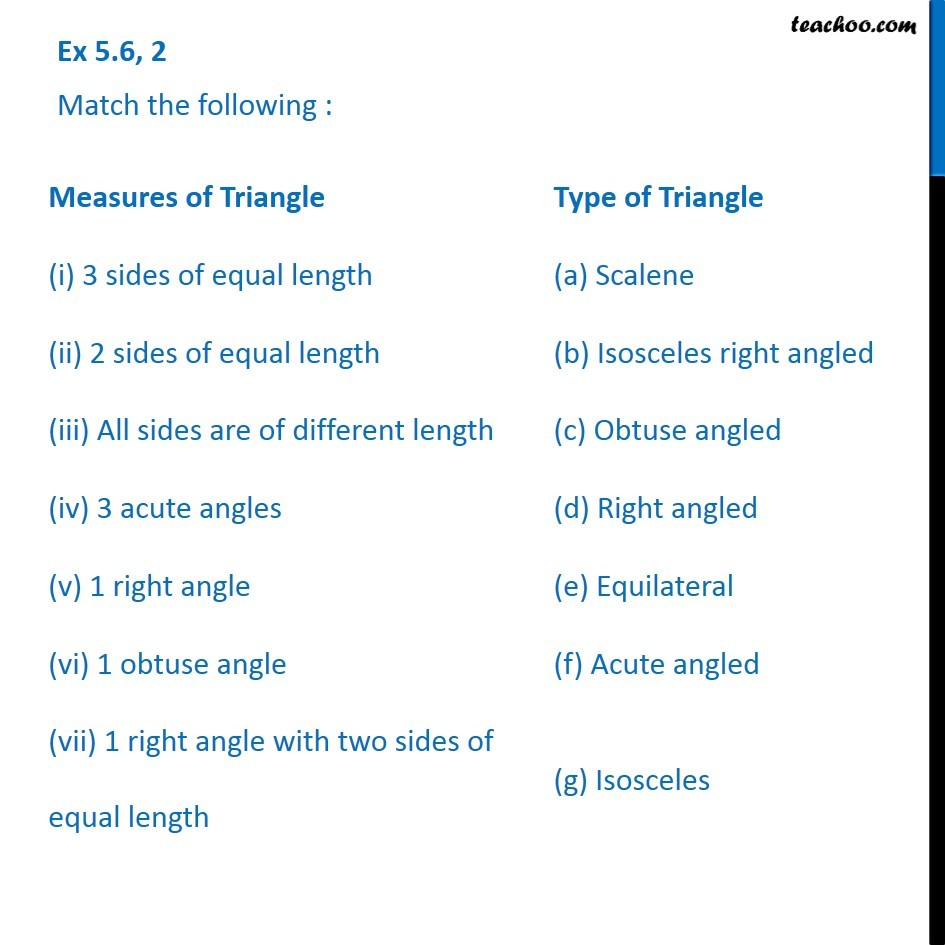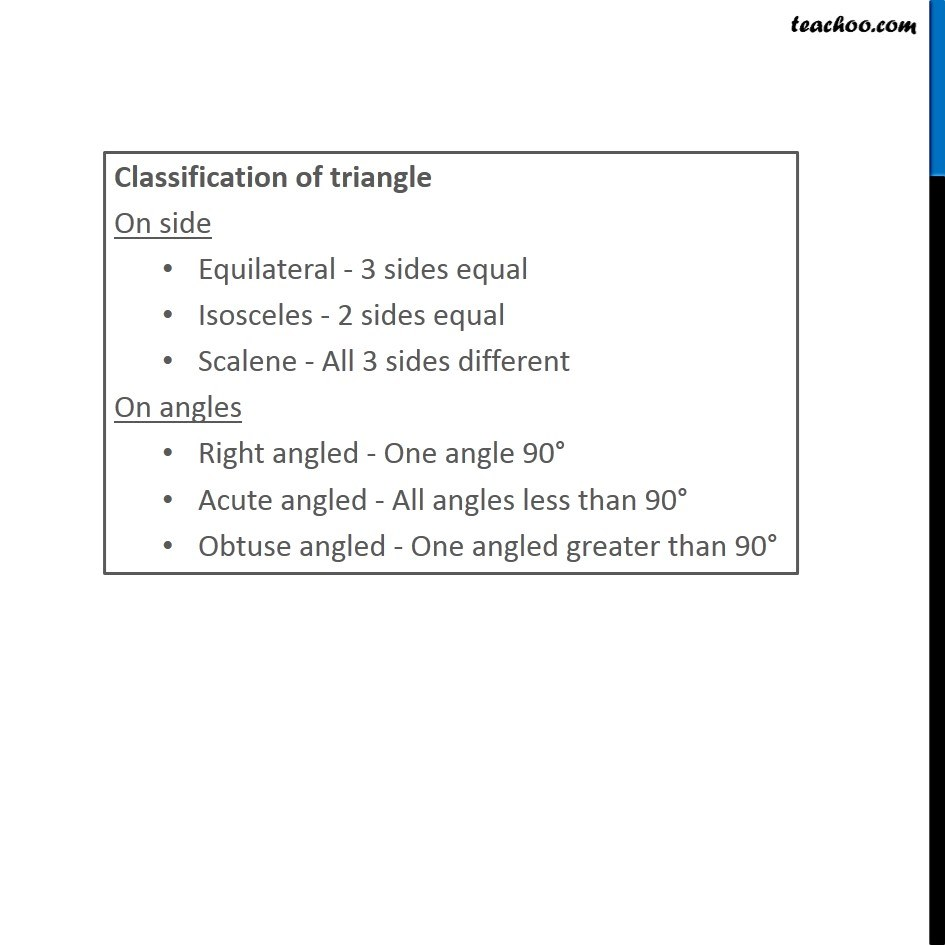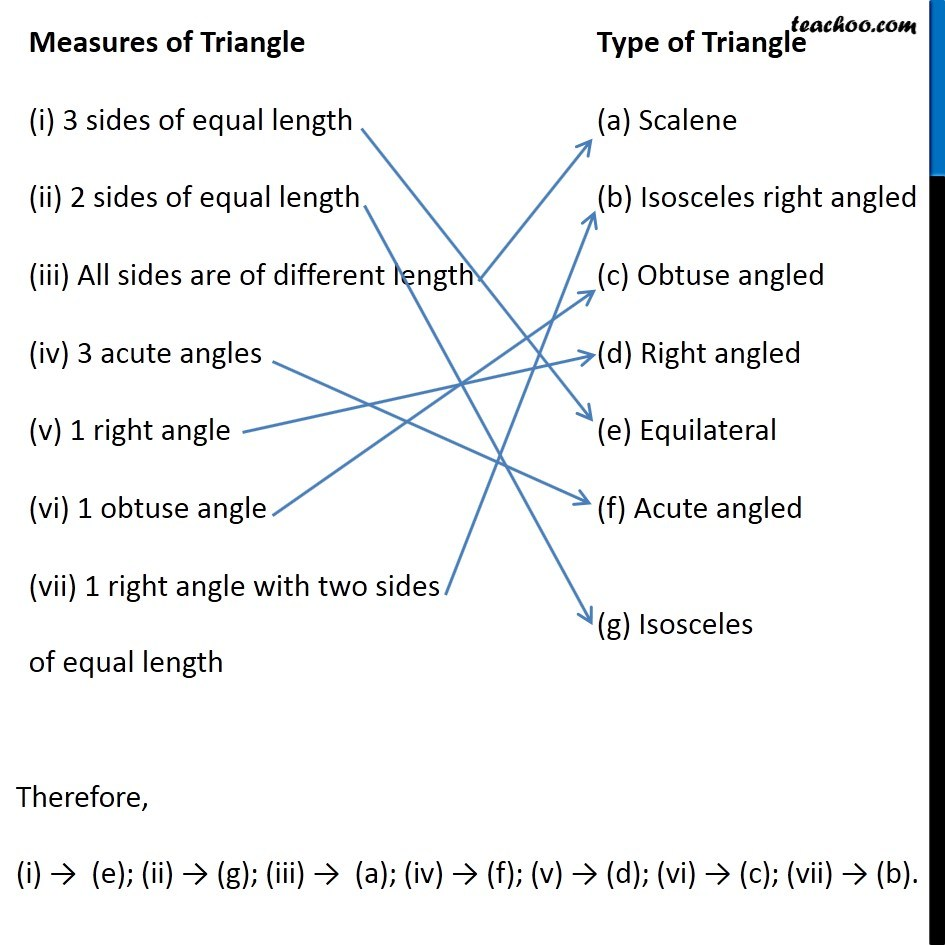1. Chapter 5 Class 6 Understanding Elementary Shapes
2. Serial order wise
3. Ex 5.6

Transcript

Ex 5.6, 2 Match the following : Classification of triangle On side Equilateral - 3 sides equal Isosceles - 2 sides equal Scalene - All 3 sides different On angles Right angled - One angle 90° Acute angled - All angles less than 90° Obtuse angled - One angled greater than 90° Therefore, (i) → (e); (ii) → (g); (iii) → (a); (iv) → (f); (v) → (d); (vi) → (c); (vii) → (b).

Ex 5.6

Chapter 5 Class 6 Understanding Elementary Shapes
Serial order wise